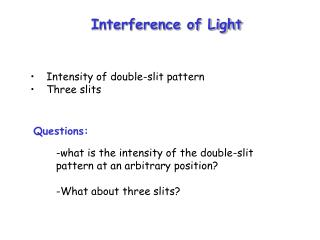DownloadDownload PresentationQuestions:

# Questions:

Download Presentation## Questions:

- - - - - - - - - - - - - - - - - - - - - - - - - - - E N D - - - - - - - - - - - - - - - - - - - - - - - - - - -
##### Presentation Transcript

1. Questions: • what is the intensity of the double-slit pattern at an arbitrary position? • What about three slits? Interference of Light • Intensity of double-slit pattern • Three slits

2. Young’s Double Slit Experiment Screen at infinity θ d Δx Path difference Δx = d sin θ d sinθ = m λ, m = 0, 1, 2, … Maximum intensity at • d sinθ = (m +½ ) λ, m = 0, 1, 2, … Minimum intensity at

3. Find the intensity of the double-slit interference pattern as a function of position on the screen. Two waves: E1 = E0sin(w t) E2 = E0sin(w t + f ) where:

4. Trigonometry: sin a + sin b = 2 cos [(a-b)/2] sin [(a+b)/2] E1 + E2 = E0[sin(w t) +sin(w t + f )] = 2 E0cos (f / 2) sin(w t + f / 2)] Resultant amplitude ER Resultant intensity, (a function of angle qto the screen)

5. I position • fringes are wide, with fuzzy edges • equally spaced (in sin θ or small θ) • equal intensity

6. Quiz With only one slit open, the intensity in the centre of the screen is 100 W/m 2. With both (identical) slits open together, the intensity at locations of constructive interference will be • zero • 100 W/m2 • 200 W/m2 • 400 W/m2

7. Example • Two narrow parallel slits are separated by 0.85mm and are illuminated by light of a 600nm wavelength. • What is the phase difference between the two interfering waves on a screen 2.8m away at a point 2.5mm from the central bright fringe? • What is the ratio of the intensity at this point to the intensity at the center of a bright fringe?

8. Three Equally-Spaced Slits θ is the phase difference between consecutive slits d Δx = dsinθ d Total,

9. Three Slits Pattern The total displacement is where = 2 (d sin )/ • When d sin = m  and , ER = 3E0 (maximum value); so the bright fringes are in the same locations as with two slits. • There are minima (zero intensity) at  = 120° and  = 240° (where d sin = /3, 2/3 ); to see this, add the first and third term above). • At  = 180° (where d sin = /2) there is a small maximum: ER = E0

10. 2 slits 4I0 I 9I0 3 slits I0 2/3 1/2 1 1/3 In wavelengths f = 2π/3 rad Δx =λ /3

11. Differences between 2-slit and 3-slit patterns: • 3 Slits: • Main peaks become narrower & brighter • One “extra” faint secondary peak in between • Main peaks ( = m2π) are in the same places As the number of slits becomes large, the main interference fringes become even narrower and brighter; the additional numerous faint peaks become less and less noticeable in comparison.

12. Many Slits (“diffraction grating”) I 0 1 -1 m=(d sin )/

13. Quiz Suppose the multiple slits from the previous slide were illuminated with white light, instead of red. What would the pattern on the screen look like? • The three bright lines would be white, with a little colour at the edges, but otherwise the same • Each of the three lines would spread into a wide coloured spectrum • Two of the three lines would spread into a wide coloured spectrum, with the centre one narrow and white